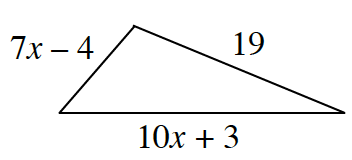Home > CC4 > Chapter 3 > Lesson 3.1.4 > Problem3-47

3-47.

The perimeter of the triangle at right is $52$ units. Write and solve an equation based on the information in the diagram. Use your solution for $x$ to calculate the length of each side of the triangle. Be sure to confirm that your answer is correct. Homework Help ✎Find the sum of the side lengths.

The sum of the side lengths is the perimeter, which we know from the problem is $52$ units.Set expression from Step 1 equal to $52$ and solve for $x$.

$(7x − 4) + (19) + (10x + 3)$

$(7x − 4) + (19) + (10x + 3) = 52$
$17x + 18 = 52$

Continue solving finding the value of $x$.

Find side lengths substituting your found value of $x$ into the expression given for each side.